1. Home
2. Examples of Standard Nonlinear Curves

# Examples of Standard Nonlinear Curves

This figure is based on Figure 3.7.1 in the The Guide to Genstat® – Part 2: Statistics. It shows the shape of curves representative of each type, although you should be aware that some curves, especially the rational functions, can vary greatly as their parameters change.

You can use this figure to help choose the type of curve to fit to your data. Click on a graph for details of the model it represents.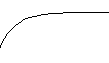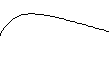Exponential (SENSE=right) Exponential (SENSE=left) Line + Exponential (SENSE=right)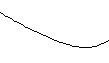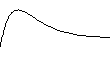Line + Exponential (SENSE=left) Critical Exponential (SENSE=right) Critical Exponential (SENSE=left)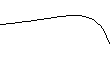Double Exponential (SENSE=right) Double Exponential (SENSE=left) Logistic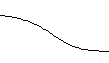Generalized Logistic Gompertz (SENSE=right, β>0) Gompertz (SENSE=left, β<0)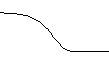Gompertz (SENSE=right, β<0) Gompertz (SENSE=left, β>0) EmaxGeneralized Emax Linear divided by Linear Quadratic divided by Linear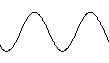Quadratic divided by Quadratic Fourier Double FourierGaussian Double Gaussian